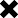### Porfolio(of different assets) return calculation.

POSTED BY ON March 12, 2013 12:12 am COMMENTS (4)

hi

I have a portfolio of different asset classes like MF, gold, real estate, FD. I know the annualized return of these indivial assets.

I would like to know the combine annualized return of the whole portfolio. OR with what rate my portfolio is growing. Here the amount invested in different asset class and their corresponding return varies

what is the formula to calculate portfolio return ?

can someone please demonstrate here with some example.

say for example.

MF annualized return for 10% (amount is 3 lacs)

FD is 8.5% ( amount is 5 lacs)

Gold is 11% (amount is 2.5 lacs)

real estate is 12% (amount is 20 lacs)

## 4 replies on this article “Porfolio(of different assets) return calculation.”

1. Dear Ainvest, you invested different amounts on different dates in different assets. But compositely all these assets are a common thing which is called your portfolio. Using the investment dates & invested amounts, as on date combined valuation of your portfolio ‘ll give you the XIRR of your portfolio. As simple as that.

Thanks

Ashal

2. ainvest says:

@Ashal

according to the XIRR documentation it is used different.(correct me if I wrong)
https://office.microsoft.com/en-us/excel-help/xirr-HP005209341.aspx

“Dates is a schedule of payment dates that corresponds to the cash flow payments. The first payment date indicates the beginning of the schedule of payments. All other dates must be later than this date, but they may occur in any order. ”

In my case, I have calculated the CAGR for each indivial asset but since the amount invested in that asset class differ I need average %age rate at which the portfolio is growing

1. You already have the CAGR (or the geometric average). From this you can calculate the weighted average return

Your total portfolio is 30.5L

So weighted average return
= 10% *(3/30.5) +8.5%*(5/30.5) + etc.

The other way is to do a blind CAGR bet two dates.
date 1 (total portfolio value)
date 2 (total portfolio value) (date 2 is say 1 year later)

then
CAGR= (Value2/Value1)-1

My guess is both ways should not differ by much. Would be great if you check and let me know.

3. Dear ainvest, please use XIRR function in your exl sheet to calculate the portfolio Return.

Thanks

Ashal

This site uses Akismet to reduce spam. Learn how your comment data is processed.